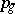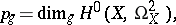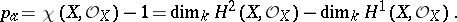# Genus of a surface

(diff) ← Older revision | Latest revision (diff) | Newer revision → (diff)
Jump to: navigation, search

A numerical birational invariant of a two-dimensional algebraic variety defined over an algebraically closed field. There are two different genera — the arithmetic genus and the geometric genus. The geometric genusof a complete smooth algebraic surfaceis equal toi.e. to the dimension of the space of regular differential-forms (cf. Differential form) on. The arithmetic genusof a complete smooth algebraic surfaceis equal toThe geometric and arithmetic genera of a complete smooth algebraic surfaceare related by the formula, whereis the irregularity of, which is equal to the dimension of the space of regular differential-forms on.